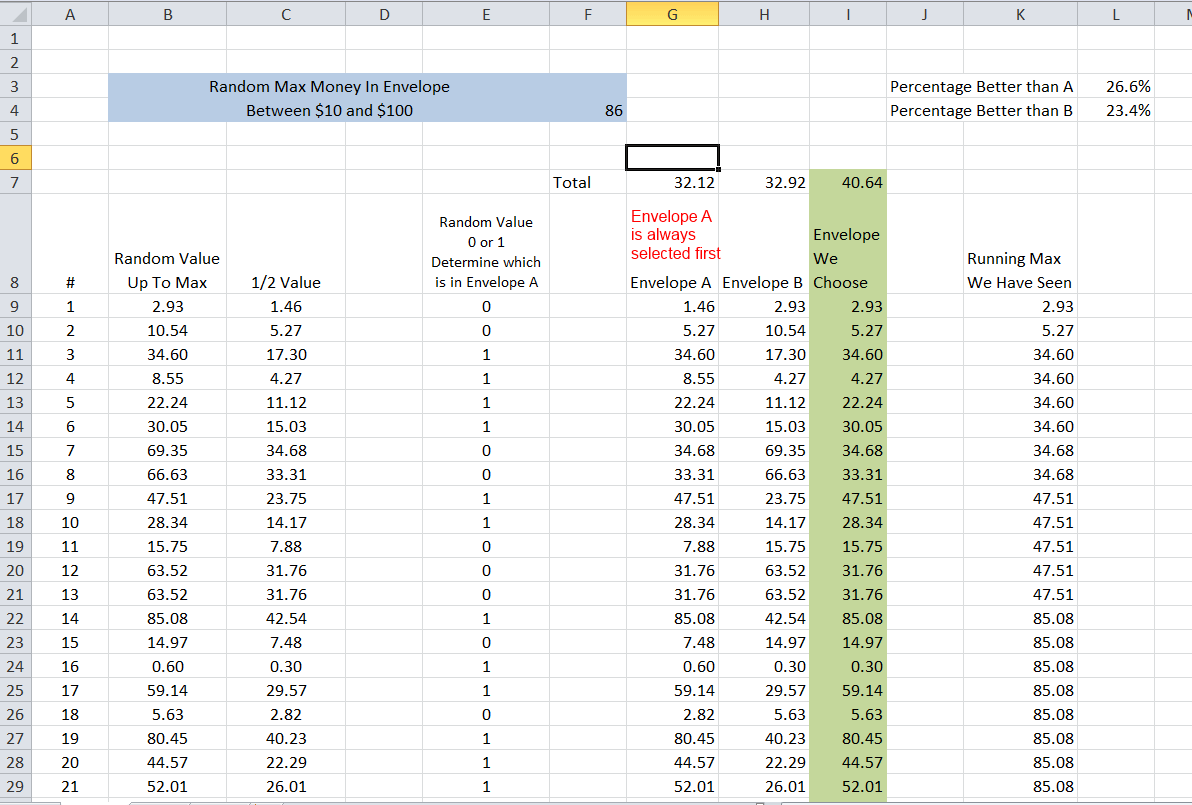# Permutations And Combinations Simplified

Permutations and combinations are an essential part of statistics. They show up in a ton of different places when you are finding the probability of anything. But it can be hard to remember the exact formula for a permutation or a combination when you need it without looking it up.   This post will show you an easy, intuitive way to understand permutations and combinations so that you only need to remember one thing, and the rest you can just calculate when you need it.

## Permutations and Combinations Basics

Permutations and combinations are a way of determining how many different possibilities of something there are.

Permutations are what you use when the order matters. For instance, if 8 people are racing in a track meet, and you want to find the different ways they could get 1st, 2nd, and 3rd place, then the order matters. So you would use a permutation.

Combinations are what you use when the order doesn’t matter.   For instance, if you have 10 different pieces of clothing you want to take on a trip, but you can only fit 7 of them in your suitcase, it matters which 7 you pick, but it doesn’t matter what order you put them in the suitcase, so you would use a combination

## The Key Point

There is one key thing to know with Permutations and Combinations, and that is the Factorial.   Typically denoted with an exclamation point !

If you want to find how many different ways you can arrange 8 different items, it is 8 Factorial, which is 8 * 7 * 6 * 5 * 4 * 3 * 2 * 1.   What this represents is that when you make your first choice of items to arrange, you have 8 to choose from. When you make your second choice, there is one less so that you have 7 to choose from, then 6 and so on.

Everything with permutations and combinations are just different applications of the Factorial.

## Permutations – Slightly Simpler Than Combinations

Let’s go back to the track example. Let’s say that you have 8 people racing on the track. The total different orders they could come in are 8 !

8 * 7 * 6 * 5 * 4 * 3 * 2 * 1 = 40320

Now let’s say you only care about the order of the first three people on the track. Clearly we would have fewer than the full 8! different permutations, because we only care about how the first 3 people finished, not all 8. So in that case you have 8 options for first place, 7 options for 2nd place, and 6 options for 3rd place, and that’s it.

8 * 7 * 6 = 336

This is the permutations of 8, choosing 3.

Now there isn’t a function that lets us just multiply 8 * 7 * 6 easily.   If we wanted the order of everyone, then 8 Factorial lets us multiply 8, down through 1, but it doesn’t stop in the middle. The way we do this is by finding 8 Factorial, and then dividing by 5 factorial. We use 5! because there are 5 items left behind that we don’t care about (8-3 = 5) That ends up beingThe

( 5 * 4 * 3 * 2 * 1)

Cancels out of both the numerator and denominator, and we are left with 8 * 7 * 6.

So to find the permutations of a subset of a group, what we have just done is

• Find the permutations of the entire group ( 8! in this case)
• Divide by the permutations of the part of the group left behind ( 5! In this case)

Why are we dividing by the permutations of the parts left behind ?   Because we don’t care what order they are in, so we need to cancel out all different orderings that they can be in.

## This is a key point to remember

• If you want to find the permutations of something, use the factorial
• If you want to find the permutations of a subset, find the permutations of the entire group, and then divide by the permutations of the set left behind.

## Combinations – Build on Permutations

• Permutations – We take the factorial of the entire set to find out the number of possibilities
• Permutations with a subset – We take the factorial of the entire set, and then divide by the factorial of the left behind set

Well for combinations we still don’t care about the order of the left behind set, but we also don’t care about the order of the set that we have chosen.   So we start with the permutations of the entire set, then divide by the permutations of the left behind set, then divide by the permutations of the chosen set.

So if we have 10 different items of clothing, and we can only choose 7 to pack, so there are 3 left behind, the number of possibilities are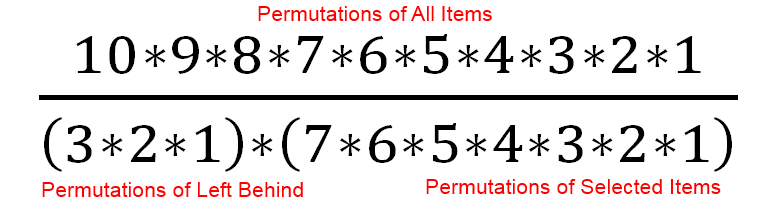Which is equal to 120

An important thing to know is that there will always be at least as many permutations of a set as combinations, and typically many more permutations than combinations.

## The Traditional Permutations & Combinations Equations

At this point, it is worth showing the traditional permutations and combinations equations. These are the things that you might typically be expected to memorize for a class, but can be challenging to remember long term

Here is the permutation equation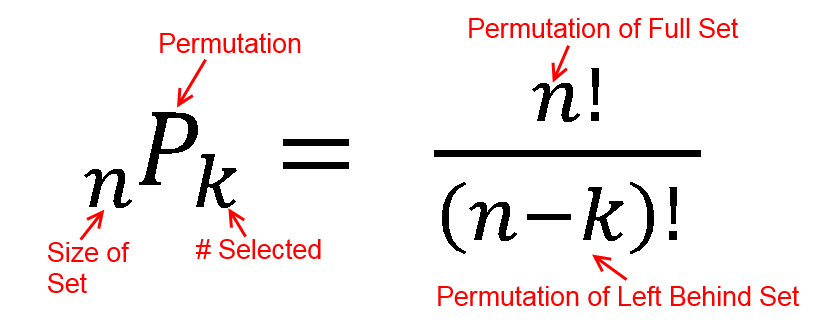And here is the combination equation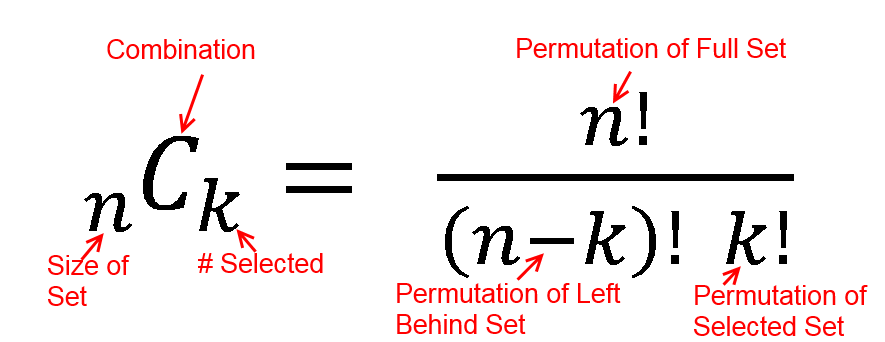So while it is manageable to memorize those equations, it is easier to just intuitively understand

• To find the permutations of a full set, take the factorial
• To find the permutations of a partial set, find the permutations of the full set, then divide by the permutations of the items left behind
• To find the combinations of a partial set, find the permutations of the full set, divide by the permutations of the items left behind, and divide by the permutations of the selected items.

## Taking It One Step Farther

If you understand using the factorial, instead of memorizing the equations, you can apply it to cases that the equations don’t cover. For example, if you have 12 items, and you need to break them up into 4 equally sized groups, how many options do you have ? (order does not matter within any group)

Once again you start with the permutations of the entire group, and then divide by the permutations of any subset whose order you don’t care about. In this case we have 4 subsets with 3 items each where the order doesn’t matter. So the equation would be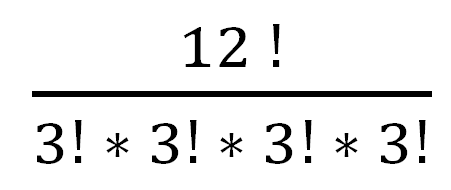Which is 19,958,400

That would be a challenging answer to get relying on just the standard equations

# Two Envelope Problem

The Two Envelope problem is one of my favorite statistical paradoxes. It is a problem that, even after you understand it, you can still revisit and be surprised by it.

## The Two Envelope Problem

You are presented with two identical envelopes. Both of the envelopes have money in them. You are told that one envelope has some amount of money in it, and the other envelope has twice as much money in it. But you don’t know how much that amount of money is. You are allowed to choose one envelope

You choose one envelope, which you decide to call Envelope A, and open it and observe X dollars. You are now given the opportunity to switch envelopes, so you calculate the expected value of switching. Since you know one envelope has twice as much money as the other, there is a 50% chance that envelope B, has twice as much as A, and a 50% chance that envelope B has half as much as A so

B = .5 * A/2 + .5 * 2A

B = 1.25 A

You conclude that the expected value of taking envelope B is 25% greater than keeping envelope A, so you start to switch. Before you do, you realize that if you had chosen envelope B to start with, you would have done the same calculation in reverse

A = .5 * B/2 + .5 * 2B

A = 1.25 B

and determined that the expected value of envelope A was 25% greater than envelope B.

How can you resolve this apparent paradox ?

## The Fairly Nerdy Solution

There are apparently quite a few solutions out there to this problem, with their authors believing their solution is clearly correct, and others disagreeing.   I’ll leave it to you to let me know what you think of my solution.

First we need to clarify how the money was put into the envelope, because it makes a big difference to the results.   The possible options are

• Coin Flip Scenario – The money is not put into the second envelope until you look at the first envelope.   i.e. if you see \$100 in the first envelope, then the game master flips a coin to decide whether to put \$200 or \$50 in the second envelope
• Infinite Money Scenario – The money is put into both of the envelopes before you choose. One envelope has X dollars and the other envelope has 2X dollars. The game master has infinite money, and would be willing to put any amount of money, including infinite money, into the envelopes
• Finite Money Scenario – The money is put into the both of the envelopes before you choose. One envelope has X dollars and the other envelope has 2X dollars.   However the game master is only willing to put a finite amount of money in the envelope, so there would be a maximum of M dollars in any envelope

Each of those options need to be considered separately, and I think that some confusion on this problem stems from not clearly defining those options

## Coin Flip Scenario

In my opinion, this scenario doesn’t really match the problem description of having two envelopes to choose from. However it does exactly match the math description of how the expected value of the second envelope is calculated.

If you are faced with this scenario, and see X dollars in your envelope, then your expected value of switching truly is 1.25 X, because the amount of money in envelope B can be calculated by

B = .5 * X/2 + .5 * 2X = 1.25X
which is how the problem is outlined.

This is basically a double or nothing bet, but instead of nothing, it’s double or half, and it is clearly a profitable bet to take.

## Infinite Money Scenario

To my mind, this is the least realistic scenario. Because while you could set up the coin flipping problem outlined above, or set up the finite money scenario outlined below, infinite money isn’t possible.   Even if you were playing the game with the richest person alive, or even the government of all of Earth, there is still some upper limit to the total amount of money. But if you do assume infinite money, what happens?

If you are assuming infinite money, the most reasonable assumption is that one envelope has some amount of money in it, between zero to infinity dollars, and the other has half that amount in it.

The first thing we notice is that the expected value of envelope A, taken all by itself, is infinity. This is because the average of all numbers between zero to infinity is infinity.   We then immediately observe that the expected value of envelope B is also infinity.   So after solving the equation of

B = .5 * A/2 + .5 * 2A

We are left with

B = 1.25 infinity

And

A = 1.25 infinity

So this scenario doesn’t really boil down to “why is the expected value of switching greater than the expected value of not switching”   it ends up being “Infinity has weird properties that don’t match our intuition”

## Finite Money Scenario

The scenario where the envelopes have finite money is the most interesting scenario in my opinion. Both because you could actually replicate it in real life, and because the math is interesting. With the finite money scenario, the most reasonable assumption for how it gets distributed is

• The game master puts a random amount of money in the first envelope, between zero dollars up to the maximum dollars he is willing to put in one envelope, denoted as M
• The game master puts half that amount of money in the second envelope
• The game master flips a fair coin to shuffle those envelopes so they are randomly presented as envelope A & envelope B

However, we are still posed with the problem, why does

B = .5 * A/2 + .5 * 2A = 1.25 A

and

A = .5 * B/2 + .5 * 2B = 1.25 B

The cause of this apparent paradox turns out to be bad intuition on the possible distribution of money in the envelopes. The assumption in this paradox is that the all dollar amounts are equally likely. Therefore you have a 50% chance of doubling your money, and a 50% chance of cutting it in half, just like in the coin flip scenario.

For that to be true, it implies a probability function that looks like this, where all numbers are equally likelyWhere M is the Maximum amount of money the game master would be willing to put in a single envelope. However the actual distribution of money in those envelopes will look like thisContrary to our assumptions, all dollar amounts are not equally likely.   There will actually be 3 times as many quantities below half of the maximum value as the number above half the max value.

So before going any farther, intuitively what will this mean ?   Well clearly the likelihood of doubling our money goes down if we have a larger amount of money.   So the equation of

B = 50% * A/2 + 50% * 2A

Doesn’t really apply if the chances of getting A/2 and 2A aren’t the same for every value of A.

Why are there more small numbers than big numbers in this distribution ?   Because of the way the money is distributed.   If we ran this many times, and randomly generated the money in the first envelope to be between 0 and M dollars over and over, and then sorted that money, we would end up with a distribution that looked like this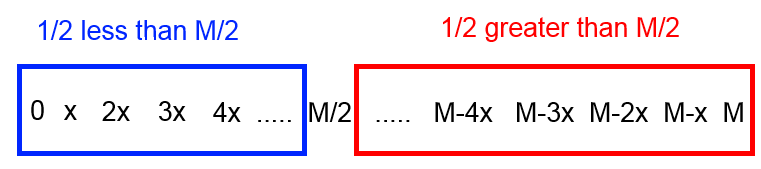Half of our numbers are less than M/2, and half are greater than M/2. Exactly what we intuitively expect.   When we generate the numbers in the second envelope by dividing all of these by 2 we end up with all of the numbers in the second envelope being less than M/2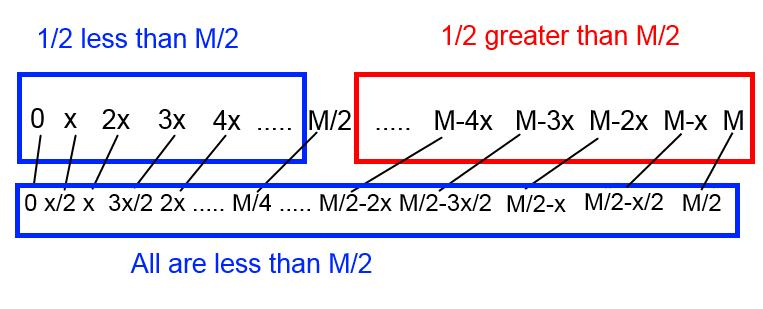In total, 1/4 of the numbers in both envelopes are greater than M/2, and 3/4 less than M/2.   Note, we set up these envelopes by putting up to a maximum amount into the first envelope and then dividing to get the second envelope. The 1/4 and 3/4 distribution would still hold if you went the other way and put up to a maximum amount into the first envelope and doubled it to get the second envelope.

At this point the intuitive solution to this paradox is clear. For any number there is not an even chance that we will double the value in the envelope vs the chance that we will cut that value in half.   The chance that we will double is larger for the small values than for the large values, which will mean that the

B = 1.25 A

A = 1.25B

Equations won’t hold.

The next section works out the math for exactly what the expected value of switching is for the finite money scenario. Then, in the final section we will exploit this information to see how if we were playing this game multiple times in a row, we actually could intelligently switch and end up with more money at the end of multiple rounds than the average expected value.

## Expected Value Of Switching – Finite Money Scenario

For calculating the expected value of switching, we will give a concrete example.   For this example, we will assume that the maximum amount of money that the game master is willing to put in an envelope is \$40.

In this scenario, the average value of the lower values is \$10, and the average value of the upper values is \$30.   The average value of all the money in the envelopes is \$15, calculated by .75 * \$10 + .25 * \$30 = \$15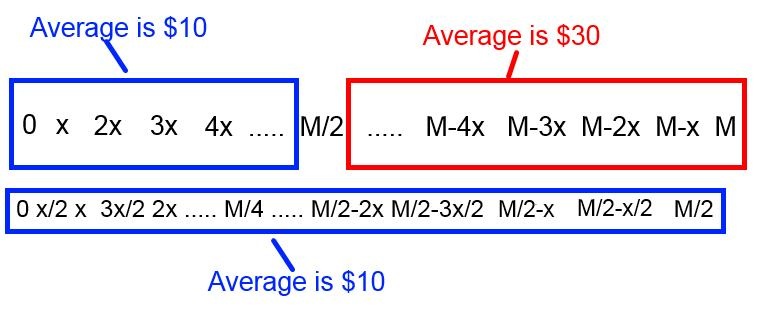At this point it’s easiest to assume that we will always get either the \$10 average value, or the \$30 average value when we open an envelope.   So when we open the first envelope, 75% of the time we will get \$10, and 25% of the time we will get \$30.So what is the expected value of switching if we have either the \$30 or the \$10 in the first envelope ?   For the \$30 it’s easy because if the first envelope has money that lies in the upper half of the range, switching will always get in the lower half of the range.   So the \$30 will always be cut in half to \$15

The \$10 is interesting because for every 3 times we see the \$10, 2 of those times we will double to \$20, and 1 time we will cut in half to \$5Breaking out the table for the expected value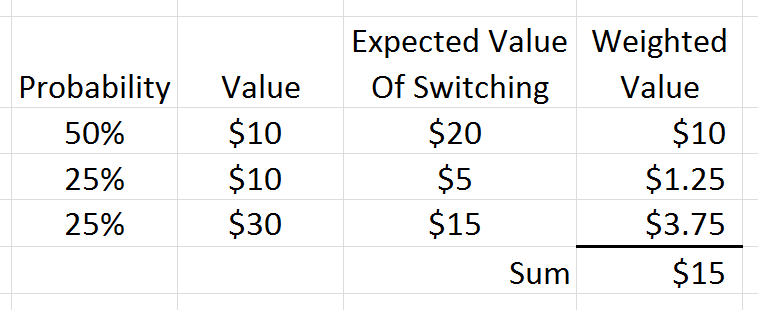Finding the weighted average of 50% of \$20, 25% of \$5, and 25% of \$15 gives us the total expected value of switching envelopes, which is \$15.

So the expected value of envelope A in this case is \$15, and if we switch, the expected value of envelope B is \$15, which are the same. This resolves our paradox, since we expect the average values in these envelopes to be the same

## Exploiting This Information

Interestingly, even though the average value of A & B are the same, this is still an exploitable game assuming:

• You play more than one round and
• You can look in your envelope before deciding whether you want to switch

Our earlier calculations show that the average value of all the switches was the same as not switching. But that was just the average value. Some of the individual switches themselves were beneficial, and some lost you money. If you can find a way to distinguish the profitable switches from the unprofitable ones, you can get more money.

The obvious solution is that you want to switch when you have the low dollar amount, and not when you have the high ones. The expected value of switching when you have the low dollar is:

• You will double your money 2 times out of 3
• You will lose half your money 1 time out of 3.On average switching when your envelope has less than half the maximum amount of money the game master would be willing to put in will yield +50% on those switches. Since you likely only have a rough guess as to how much money could be in the envelopes, a good (not necessarily perfect) strategy would be to switch envelopes the first time, and then after that switch if the amount in your envelope is less than half of the running maximum you have seen.

I simulated that strategy in the Excel below, playing 1000 games with a random maximum amount of money that could be in the envelope, random amounts in one envelope, half that amount in the other, randomly shuffled.   Whatever envelope got selected (from the random shuffle) we call envelope A, and the other envelope gets call B

Implementing that strategy gave approximately 25% more money than just picking the A or B envelopes.   You can download that Excel file here.